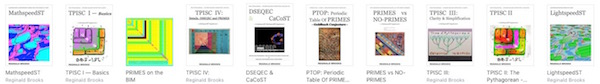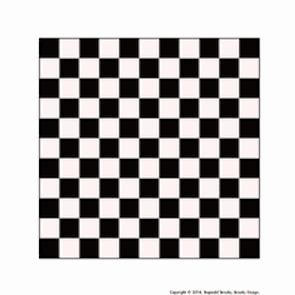# PRIMES vs NO-PRIMES: Two definitive methods for revealing all the PRIME numbers ~A MathspeedST Supplement~Statement

Gather the NO-PRIMES

6yx+-y

Remains are the PRIMES

How can a deceptively simple matrix grid square give us:

1. Inverse Square Law (ISL);

2. Pythagorean Triples (PTs);

3. Primes (P) vs. No-Primes (NP)?

The BIM (BBS-ISL Matrix) does that!

What started as the invention/discovery of the ISL matrix — a simple grid of natural Whole Integer Numbers (WIN) — has led to an unforeseen connection between the ISL — PPTs — PRIMES.

The BIM is an infinitely expanding matrix where every cell within the grid is uniquely occupied by a WIN that is itself simply the difference between its horizontal and vertical intersection values of the main, Prime Diagonal (PD).

The PD mirror divides the grid into symmetrical halves. The PD is key as it consists solely of the squares of the Axis numbers (1,2,3,...) as A2 = PD.

In fact, drop vertically down from any PD squared number until you intersect with its squared complement and you will have landed on a Primitive Pythagorean Triple (PPT) row, with a2 + b2 culminating with c2 where that row now intercepts horizontally with the PD, giving a2 + b2 = c2.

Repeating this will identify all the PPTs.

But wait! Now divide every Inner Grid (IG) cell value by 24 and you will generate a criss-crossing diamond grid overlay pattern with almost magical properties!

The BIM÷24 grid will break out the overall matrix into two (2) types of ODD Axis rows:

• those ÷3 that are never PPT, never PRIMES = Non-Active Rows (NON-AR);

• those NOT ÷3 that PPTs and PRIMES are

exclusively found on = Active Row Sets (ARS) or Active Rows (AR) for short. NOTE: a necessary, but not sufficient, condition as some AR have neither.

The reason the ARS is plural is because there are two ODD Axis rows — AR — in each ARS.

These two (2) ODD Axis AR alternate with an ODD Axis NON-AR giving the (NON-AR)—AR—AR—(NON-AR)—AR—AR—(NON-AR)... pattern. Basically for every ÷3 row, one has two NOT ÷3 rows.

This becomes important as the ÷3/NOT ÷3 pattern is key to identifying the Number Pattern Sequence (NPS) of ALL the NO-PRIMES (NP) and that NPS ultimately defines the elusive pattern of the PRIMES as: ALL ODD WIN (≧3) - NP = P.

This has culminated — at least at this point in this exciting mathematical journey — in two (2) simple methods for generating ALL the NP:

1. Algebraic: *NP = 6yx ± y  let y = ODD WIN, x = 1,2,3,... (*one must add the exponentials of 3, 3x, if one does not first eliminate all ÷3 WIN).

2. Algebraic Geometry: where it all began on the BIM by subtracting (eliminating) ALL the **NP that are shape-located directly on the BIM, one can reveal the remaining PRIMES (**here, one must include the ODD Axis2 values).

Both methods nicely dovetail directly on the BIM.

*NP = 6yx ± y is the simple, algebraic equation that is derived from and summarizes the visualization approach we see directly on the BIM. This dovetailing of the pure algebra approach with the pure geometry of the BIM gives us the algebraic geometry method. Visual thinkers like to "see" the algebra and algebraic geometry at work — play!

The regular BIM by itself contains all the same information for either method. The NPS of the algebraic and algebraic geometry methods pops out more readily when we do a minor alteration to the BIM — squaring the Axis numbers. Since we are only working with the ODD numbers, this means simply squaring the ODD Axis numbers. And to completely correlate the *NP = 6yx ± y  algebraic method to the BIM, we need to manually add the exponentials of 3 (3x) to the listings (including those in spreadsheet table format) of the NP numbers generated by the equation. That's it!

Looking at the BIM's lower symmetry — after eliminating all the EVEN numbers and squaring the ODD Axis numbers — a clear NPS of ALL the NP is revealed. A L-shaped-double-wide-diagonal-path pattern that corresponds systematically, step-by-step with algebraic solutions of *NP = 6yx ± y and the pre geometry-shape visualization of the algebraic geometry method. Both methods give the same identical NP results because each is simply the same logical process only coming at it from two different approaches.

Now the really interesting part of all of this is all the NPS revealed in the exposure of ALL of the NP is itself simply the converse — inverse — of ALL the PRIMES.

One — take your pick — is simply the negative space of the other. If we call the PRIMES the positive space, then their NPS is revealed by the negative space NO-PRIMES!

When visualizing the PRIMES vs. NO-PRIMES on the BIM, it is much easier to "see" the NP as the positive space with all the NPS and the PRIMES as the remaining negative space — a space completely defined by that of its opposite (inverse) NO-PRIMES space as: NP + P = ALL ODD numbers (≧3).

Yes, a deceptively simple matrix grid square (BIM) can give us:

1. Inverse Square Law (ISL);

2. Pythagorean Triples (PTs);

3. Primes (P) vs. No-Primes (NP)!

~~~

This is an additional supplement to the MathspeedST ebook. Other titles include The Pythagorean-Inverse Square Connection (TPISC):

TPISC I: Basics

TPISC III: Clarity and the Tree of Primitive Pythagorean Triples

TPISC IV: Details, DSEQEC and PRIMES.

Excerpt From: Reginald Brooks. “PRIMES vs NO-PRIMES...” iBook.

Platforms: This is a multi-touch, interactive ebook made with iBooks Author and published in the Apple iBookstore. Currently, it requires an iPad, or OS X running on a Mac, and the free iBooks app to download and read.

Most of the same content is found online here on my website in the form of white papers and multi-media. HERE is the Media Center link.

Selected SCREENSHOTS for PRIMES vs NO-PRIMES

(NOTE: iBooks are available for reading on your Mac or iOS device.)

Artist Link in iTunes iBookstore: Reginald Brooks~---

~ MathspeedST: The Ubiquitous Information of Numbers white paper
~ LightspeedST: Leapfrogging @ The Speed of Light
T H E   U B I Q U I T O U S   I N F O R M A T I O N    O F    N U M B E R S new media net.art project
~ MathspeedST: Leapfrogging Lightspeed FASTER than The Speed of Light MOVIES
The contents of MathspeedST and LightspeedST, as well as most of the TPISC Series, can be viewed as White Papers in the Art Theory 101 links below.

Relevant White Papers by the Author

http://www.brooksdesign-ps.net/Reginald_Brooks/Code/Html/arthry5.htm

titles and topics:

### LightspeedST: The Complete New Media net.art project (HOME)

KEYWORDS TAGS:
PRIMES, NO-PRIMES, Periodic Table Of Primes (PTOP) and the Goldbach Conjecture, BIM, algebraic, algebraic geometry, Active Row Sets, AR, ARS, NON-Active Row, Number Pattern Sequence, NPS, TPISC, The Pythagorean - Inverse Square Connections, Pythagorean Triangles, Pythagorean Triples, primitive Pythagorean Triples, non-primitive Pythagorean Triples, Pythagorean Theorem, Pythagorus Theorem, The Dickson Method, BBS-ISL Matrix, Expanded Dickson Method, r-sets, s-set, t-sets, Pair-sets, geometric proofs, MathspeedST, leapfrogging LightspeedST FASTER than the speed of light, Brooks (Base) Square- Inverse Square Law (ISL), BBS-ISL matrix grid, The Architecture Of SpaceTime (TAOST), The Conspicuous Absence Of Primes (TCAOP), A Fresh Piece Of Pi(e), AFPOP, Numbers of Inevitability,LightspeedST, Teachers, Educators and Students (TES), number theory, ubiquitous information, FASTER than the speed of light, primes, prime numbers, fractals, mathematics, Universe, cosmos, patterns in number.

Art Theory 101 / White Papers Index
 PIN: Pattern in Number...from primes to DNA. | PIN: Butterfly Primes...let the beauty seep in. | PIN: Butterfly Prime Directive...metamorphosis. | PIN: Butterfly Prime Determinant Number Array (DNA) ~conspicuous abstinence~. | GoDNA: the Geometry of DNA (axial view) revealed. | SCoDNA: the Structure and Chemistry of DNA (axial view). | The LUFE Matrix | The LUFE Matrix Supplement | The LUFE Matrix: Infinite Dimensions | The LUFE Matrix: E=mc2 | Dark Matter=Dark Energy | The History of the Universe in Scalar Graphics | The History of the Universe_update: The Big Void | Quantum Gravity ...by the book | The Conservation of SpaceTime | LUFE: The Layman's Unified Field Expose` | GoMAS: The Geometry of Music, Art and Structure ...linking science, art and esthetics. Part I | Brooks (Base) Square (BBS): The Architecture of Space-Time (TAOST) and The Conspicuous Absence of Primes (TCAOP) - a brief introduction to the series net.art index | netart01: RealSurReal...aClone, 2001 | netart02: Hey!Ufunk'n with my DNA? | netart03: 9-11_remembered | netart04: Naughty Physics (a.k.a. The LUFE Matrix) | netart05: Your sFace or Mine? | netart06: Butterfly Primes | netart07: Music-Color-ISL | netart08: BBS-ISL matrix | netart09: BBS-interactive (I) | netart10: Sunspots and Solar Flares | netart11: Music-Color-ISL (II-III) | Art Theory 101: PIN, DNA, LUFE Matrix, GoMAS, BBS index | home Copyright 2019, Reginald Brooks, Brooks Design. All rights reserved. iTunes, iTunes Store, Apple Books, iBooks, iBooks Store, iBooks Author, Mac OS are registered® trademarks of Apple Inc. and their use on this webpage does not reflect endorsement by Apple Inc.